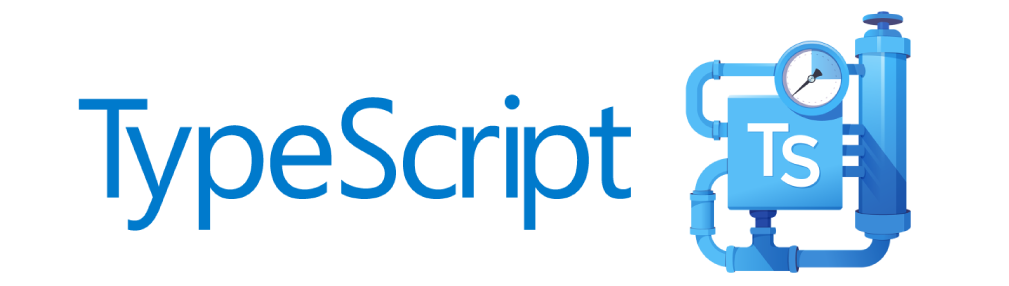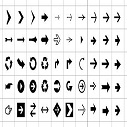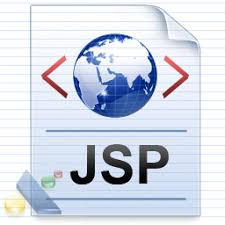# When the NOCOPY Hint is Ignored PL/SQL

The NOCOPY hint can help improve performance of subprograms by avoiding the copying of values of IN OUT arguments, but there are a number of circumstances under which the compiler will ignore the NOCOPY hint.

The NOCOPY compiler hint may be useful for improving performance of PL/SQL subprogram calls that pass "large" structures (such as collections with many elements or records with many fields) as IN OUT or OUT arguments. These types of arguments (without NOCOPY) are passed by value, which means that the PL/SQL runtime engine will make a copy of the contents of the argument twice. NOCOPY asks the compiler to avoid the copying by passing by reference, which means that any changes made to the argument in the subprogram take effect immediately and are not "reversed" if the subprogram terminates with an unhandled exception.

Our inclusion of the NOCOPY hint is only a request. There are a number of scenarios that cause the compiler to ignore the hint and continue to pass the argument by value. These include:

• The actual parameter must be implicitly converted to the data type of the formal parameter.
• The actual parameter is the element of an associative array.
• The actual parameter is a scalar variable with the NOT NULL constraint.
• The actual parameter is a scalar numeric variable with a range, size, scale, or precision constraint.
• The actual and formal parameters are records, one or both was declared with %ROWTYPE or %TYPE, and constraints on corresponding fields differ.
• The actual and formal parameters are records, the actual parameter was declared (implicitly) as the index of a cursor FOR LOOP statement, and constraints on corresponding fields differ.
• The subprogram is invoked through a database link or as an external subprogram.

In all of these cases, the NOCOPY hint is silently ignored by the PL/SQL compiler and the IN OUT argument's value is passed to the procedure by value (the default), instead of being passed by reference. As a result, if the subprogram call ends with an unhandled exception, the value of the argument IS NOT changed when the subprogram exits.

### Example :

```CREATE OR REPLACE PROCEDURE plch_proc (
p1 IN NUMBER, p2 IN OUT NOCOPY NUMBER )
AS
BEGIN
p2 := p1 + p2 ;

RAISE PROGRAM_ERROR;
END;
/

DECLARE
a  NUMBER NOT NULL := 10 ;
b  NUMBER NOT NULL := 90 ;
BEGIN
BEGIN
plch_proc (a, b);
EXCEPTION
WHEN OTHERS THEN NULL;
END;

DBMS_OUTPUT.put_line ('Result is '|| b);
END;

Result :

The NOT NULL constraint on variable "b" prevents the NOCOPY hint to be followed by the compiler. The output displayed is "Result is 90", same as without NOCOPY.

DECLARE
a  NUMBER(3) := 10 ;
b  NUMBER(3) := 90 ;
BEGIN
BEGIN
plch_proc (a, b);
EXCEPTION
WHEN OTHERS THEN NULL;
END;

DBMS_OUTPUT.put_line ('Result is '|| b);
END;

Result :

The NUMBER size constraint on variable "b" prevents the NOCOPY hint to be followed by the compiler. The output displayed is "Result is 90", same as without NOCOPY.

DECLARE
TYPE t_number IS TABLE OF NUMBER INDEX BY PLS_INTEGER;
l_numbers  t_number;
BEGIN
l_numbers(10) := 10;
l_numbers(90) := 90;

BEGIN
plch_proc ( l_numbers(l_numbers.FIRST),
l_numbers(l_numbers.LAST) );
EXCEPTION
WHEN OTHERS THEN NULL;
END;

DBMS_OUTPUT.put_line ('Result is '|| l_numbers(l_numbers.LAST) );
END;

Result :

Individual elements of collections cannot be passed by reference, so the NOCOPY hint has no effect. The output displayed is "Result is 90", same as without NOCOPY. A collection as a whole, however, can be passed by reference using the NOCOPY compiler hint.

DECLARE
a  VARCHAR2(5) := '10' ;
b  VARCHAR2(5) := '90' ;
BEGIN
BEGIN
plch_proc (a, b);
EXCEPTION
WHEN OTHERS THEN NULL;
END;

DBMS_OUTPUT.put_line ('Result is '|| b);
END;

Result :

The NOCOPY hint is ignored when an implicit conversion is required between the datatype of the actual argument ( VARCHAR2(5) ) and that of the formal argument ( NUMBER ). The output displayed is "Result is 90", same as without the NOCOPY hint.
Each of the incorrect choices represents a sample case for one of the restrictions on the use of NOCOPY, which means that this hint will be ignored. Since the subprogram call ends with an unhandled PROGRAM_ERROR, in each of these cases the variable passed to the NOCOPY argument will preserve its initial value, assigned before the subprogram call.

DECLARE
a  NUMBER := 10 ;
b  NUMBER := 90 ;
BEGIN
BEGIN
plch_proc (a, b);
EXCEPTION
WHEN OTHERS THEN NULL;
END;

DBMS_OUTPUT.put_line ('Result is '|| b);
END;

Result :

NOCOPY will be used for an unconstrained NUMBER variable, so this block correctly displays "Result is 100".
In the case of the correct choice, variables "a" and "b" are defined as NUMBER, without any constraints, and thus the NOCOPY hint will be obeyed by the compiler. As a result, the value of the IN OUT argument "p2" will be changed immediately  by the first assignment statement in the subprogram and this change remains visible when the subprogram exits, regardless of the same unhandled PROGRAM_ERROR exception.
```Admin on Nov 09, 2019 at 12:05 am

If you like dEexams.com and would like to contribute, you can write your article here or mail your article to admin@deexams.com . See your article appearing on the dEexams.com main page and help others to learn.

#### Post Comment

##### WEB TECHNOLOGY##### TypeScript Programmning Language##### New HTML5 APIs##### HTML Arrow -> Range: Decimal 8592-8703. Hex 2190-21FF.##### How To Override One CSS Class With Another×